Clocks and curvature

Next: Photographic polyhedron Up: Geometry and the Imagination Previous: The celestial image

Clocks and curvature

The total curvature of any surface topologically equivalent to the sphere is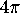. This can be seen very simply from the definition of the curvature of a region in terms of the angle of rotation when the surface is rolled around on the plane; the only problem is the perennial one of keeping proper track of multiples of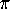when measuring the angle of rotation. Since are trying to show that the total curvature is a specific multiple of, this problem is crucial. So to begin with let's think carefully about how to reckon these angles correctly

Clocks

Suppose we have a number of clocks on the wall. These clocks are good mathematician's clocks, with a 0 up at the top where the 12 usually is. (If you think about it, 0 o'clock makes a lot more sense than 12 o'clock: With the 12 o'clock system, a half hour into the new millennium on 1 Jan 2001, the time will be 12:30 AM, the 12 being some kind of hold-over from the departed millennium.)

Let the clocks be labelled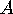,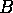,, .... To start off, we set all the clocks to 0 o'clock. (little hand on the 0; big hand on the 0), Now we set clockahead half an hour so that it now the time it tells is 0:30 (little hand on the 0 (as they say); big hand on the 6). What angle does its big hand make with that of clock? Or rather, through what angle has its big hand moved relative to that of clock? The angle is. If instead of degrees or radians, we measure our angles in revs (short for revolutions), then the angle is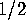rev. We could also say that the angle ishour: as far as the big hand of a clock is concerned, an hour is the same as a rev.

Now take clockand set it to 1:00. Relative to the big hand of clock, the big hand ofhas moved through an angle of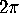, or 1 rev, or 1 hour. Relative to the big hand of, the big hand ofhas moved through an angle of, orrev. Relative to the big hand of, the big hand ofhas moved through an angle of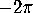, or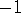rev, and the big hand ofhas moved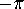, orrev.

Curvature

Now let's describe how to find the curvature inside a disk-like region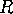on a surface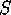, i.e. a region topologically equivalent to a disk. What we do is cut a small circular band running around the boundary of the region, cut the band open to form a thin strip, lay the thin strip flat on the plane, and measure the angle between the lines at the two end of the strip. In order to keep the's straight, let us go through this process very slowly and carefully.

To begin with, let's designate the two ends of the strip as the left end and the right end in such a way that traversing the strip from the left end to the right end corresponds to circling clockwise around the region. We begin by fixing the left-hand end of the strip to the wall so that the straight edge of the cut at the left end of the strip-the cut that we made to convert the band into a strip-runs straight up and down, parallel to the big hand of clock, and so that the strip runs off toward the right. Now we move from left to right along the strip, i.e. clockwise around the boundary of the region, fixing the strip so that it lies as flat as possible, until we come to the right end of the strip. Then we look at the cut bounding the right-hand end of the strip, and see how far it has turned relative to the left-hand end of the strip. Since we were so careful in laying out the left-hand end of the strip, our task in reckoning the angle of the right-hand end of the strip amounts to deciding what time you get if you think of the right-hand end of the strip as the big hand of a clock. The curvature inside the region will correspond to the amount by which the time told by the right-hand end of the strip falls short of 1:00.

For instance, say the regionis a tiny disk in the Euclidean plane. When we cut a strip from its boundary and lay it out as described above, the time told by its right hand end will be precisely 1:00, so the curvature ofwill be exactly 0. Ifis a tiny disk on the sphere, then when the strip is laid out the time told will be just shy of 1:00, say 0:59, and the curvature of the region will be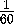rev, or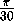.

When the regionis the lower hemisphere of a round sphere, the strip you get will be laid out in a straight line, and the time told by the right-hand end will be 0:00, so the total curvature will be 1 rev, i.e.. The total curvature of the upper hemisphere isas well, so that the total curvature of the sphere is.

Another way to see that the total curvature of the sphere isis to take as the regionthe outside of a small circle on the sphere. When we lay out a strip following the prescription above, being sure to traverse the boundary of the regionin the clockwise sense as viewed from the point of view of the region, we see that the time told by the right hand end of the strip is very nearlyo'clock! The precise time will be just shy of this, say:59, and the total curvature of the region will then be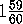revs. Taking the limit, the total curvature of the sphere is 2 revs, or.

But this last argument will work equally well on any surface topologically equivalent to a sphere, so any such surface has total curvature.

Where's the beef?

This proof that the total curvature of a topological sphere isgives the definite feeling of being some sort of trick. How can we get away without doing any work at all? And why doesn't the argument work equally well on a torus, which as we know should have total curvature 0? What gives?

What gives is the lemma that states that if you take a disklike regionand divide it into two disklike subregions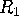and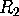, then the curvature insidewhen measured by laying out its boundary is the sum of the curvatures insideandmeasured in this way. This lemma might seem like a tautology. Why should there be anything to prove here? How could it fail to be the case that the curvature inside the whole is the sum of the curvatures inside the parts? The answer is, it could fail to be the case by virtue of our having given a faulty definition. When we define the curvature inside a region, we have to make sure that the quantity we're defining has the additivity property, or the definition is no good. Simply calling some quantity the curvature inside the region will not make it have this additivity property. For instance, what if we had defined the curvature inside a region to be, no matter what the region? More to the point, what if in the definition of the curvature inside a region we had forgotten the proviso that the regionbe disklike? Think about it.

Next: Photographic polyhedron Up: Geometry and the Imagination Previous: The celestial image

Peter Doyle